Скачать презентацию 5 8 What Is The Area Pg 27

ee7562e7253c2f8cae3eca05b01f4faf.ppt

• Количество слайдов: 26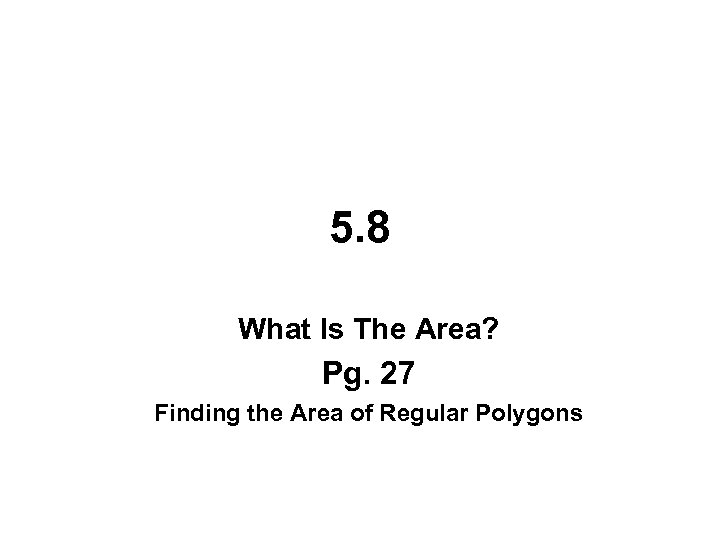5. 8 What Is The Area? Pg. 27 Finding the Area of Regular Polygons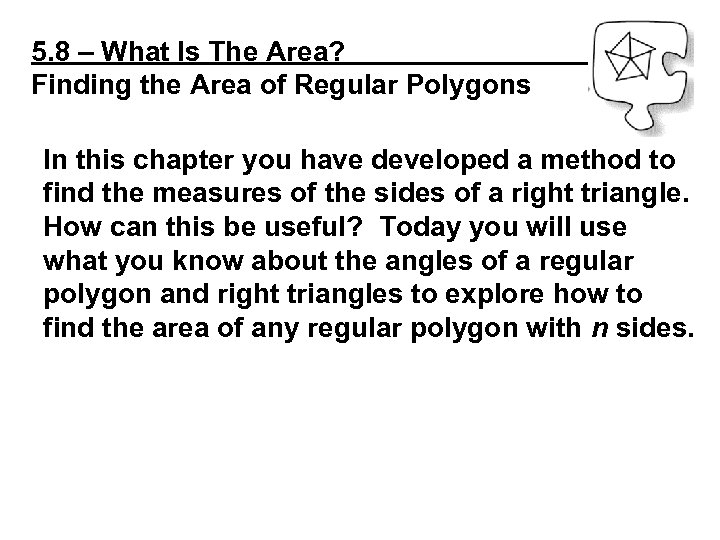5. 8 – What Is The Area? Finding the Area of Regular Polygons In this chapter you have developed a method to find the measures of the sides of a right triangle. How can this be useful? Today you will use what you know about the angles of a regular polygon and right triangles to explore how to find the area of any regular polygon with n sides.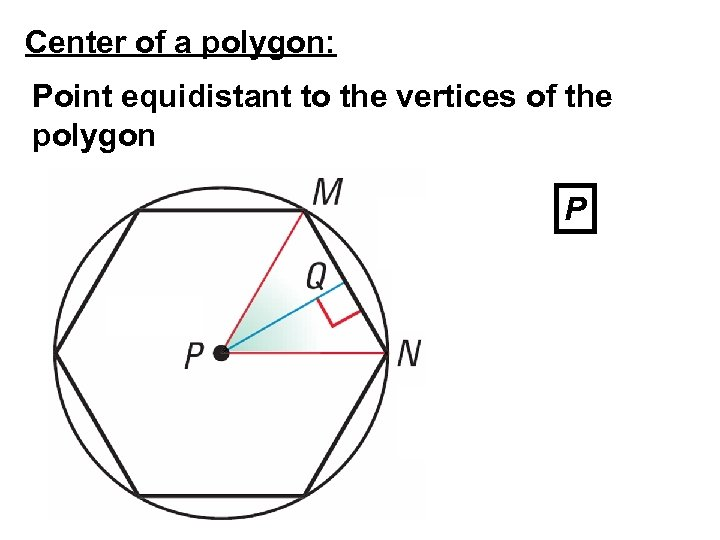Center of a polygon: Point equidistant to the vertices of the polygon P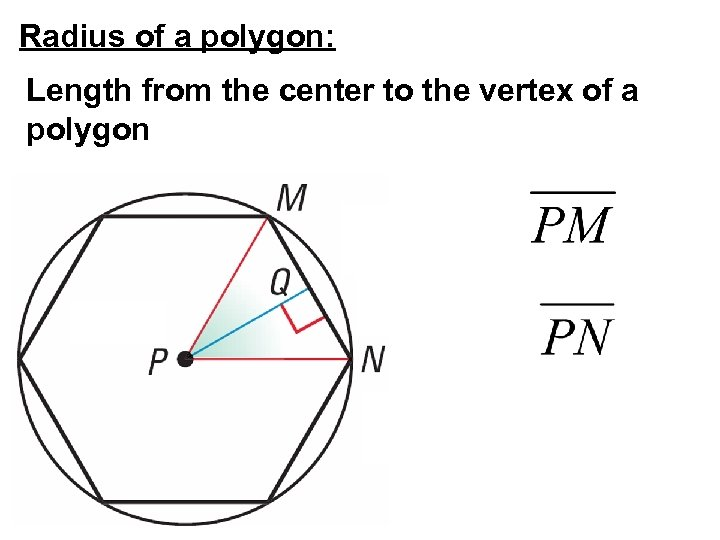Radius of a polygon: Length from the center to the vertex of a polygon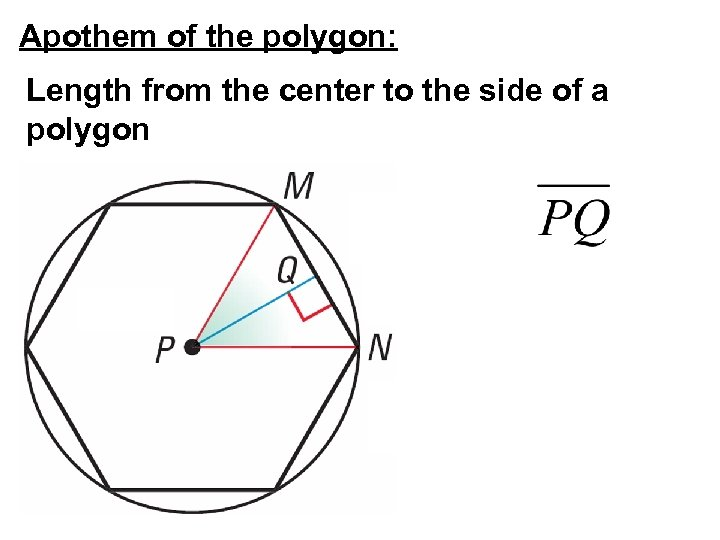Apothem of the polygon: Length from the center to the side of a polygon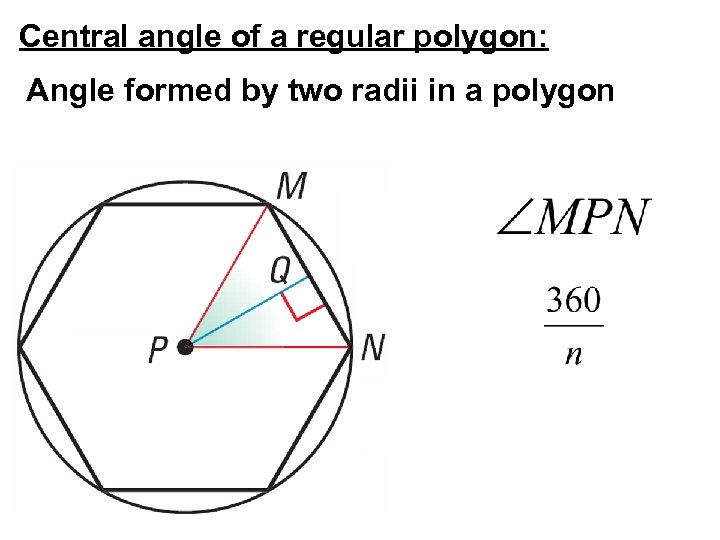Central angle of a regular polygon: Angle formed by two radii in a polygon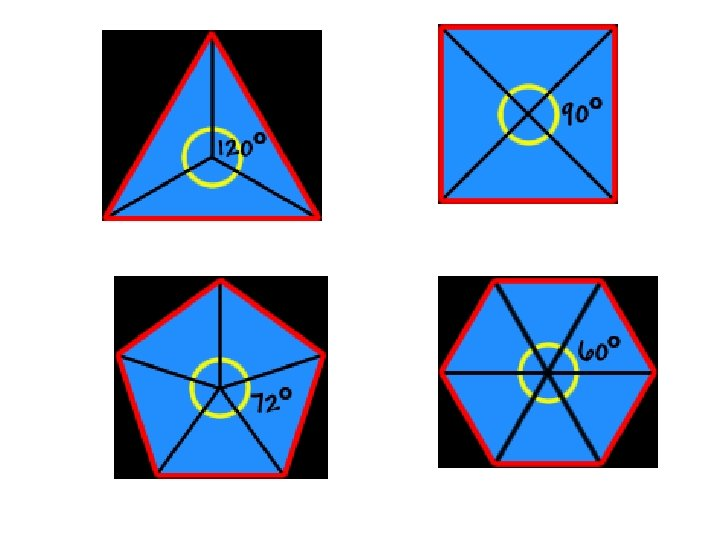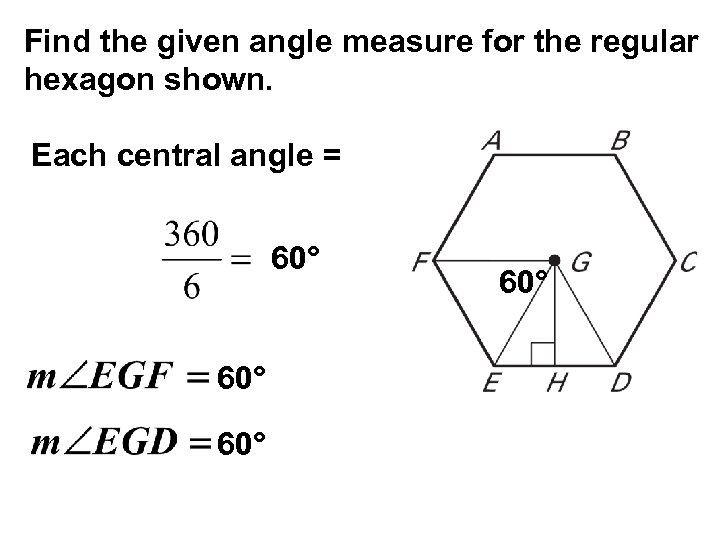Find the given angle measure for the regular hexagon shown. Each central angle = 60° 60°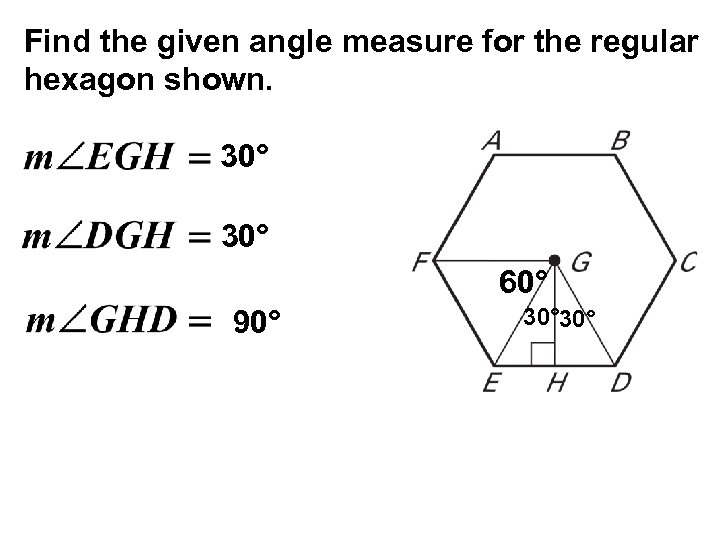Find the given angle measure for the regular hexagon shown. 30° 60° 90° 30°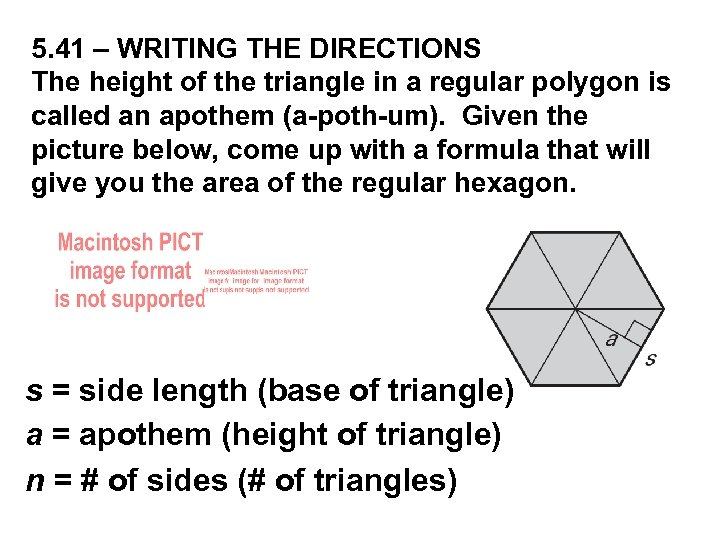5. 41 – WRITING THE DIRECTIONS The height of the triangle in a regular polygon is called an apothem (a-poth-um). Given the picture below, come up with a formula that will give you the area of the regular hexagon. s = side length (base of triangle) a = apothem (height of triangle) n = # of sides (# of triangles)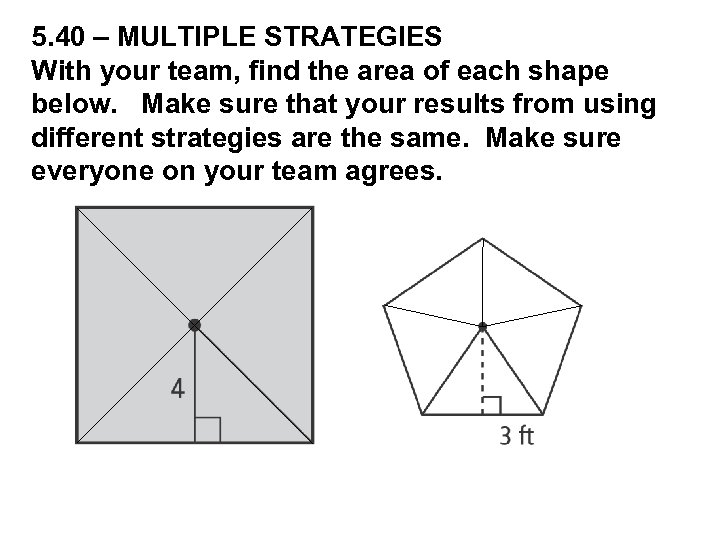5. 40 – MULTIPLE STRATEGIES With your team, find the area of each shape below. Make sure that your results from using different strategies are the same. Make sure everyone on your team agrees.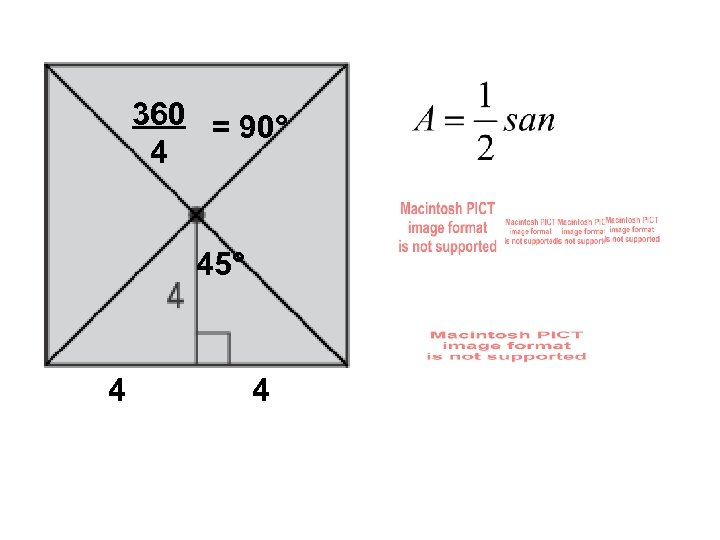360 = 90° 4 45° 4 4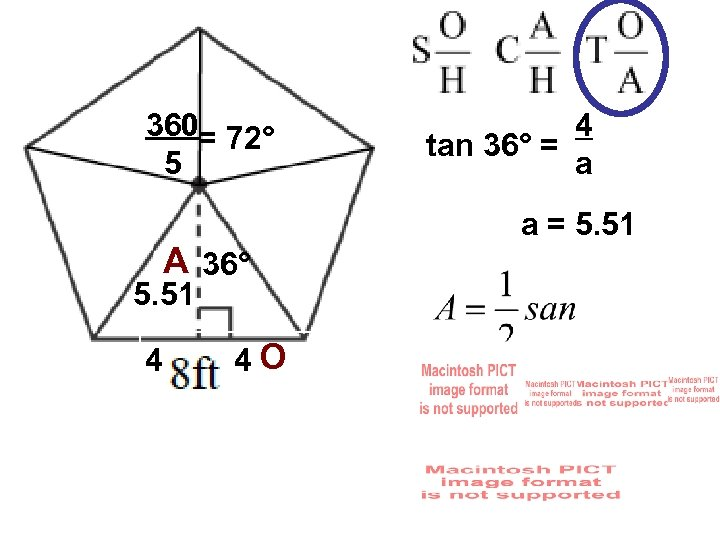360= 72° 5 A 36° 5. 51 4 4 O 4 tan 36° = a a = 5. 51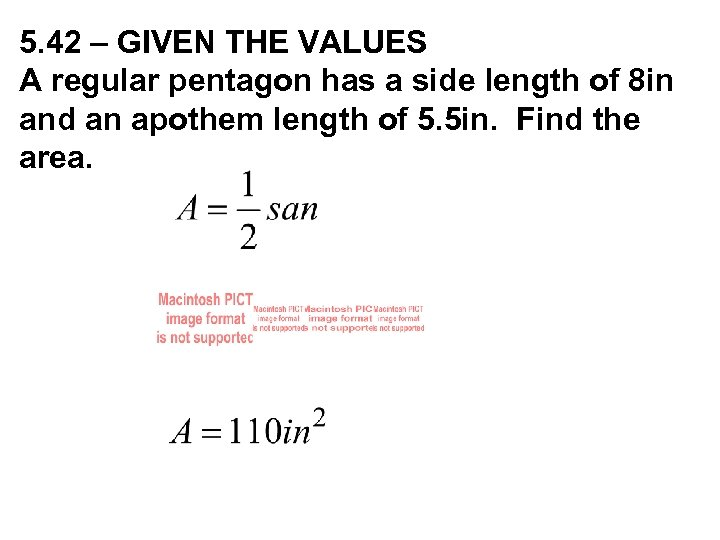5. 42 – GIVEN THE VALUES A regular pentagon has a side length of 8 in and an apothem length of 5. 5 in. Find the area.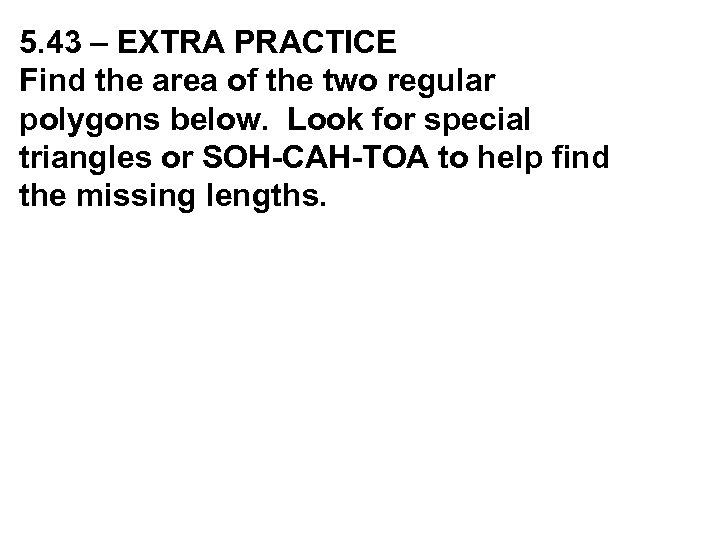5. 43 – EXTRA PRACTICE Find the area of the two regular polygons below. Look for special triangles or SOH-CAH-TOA to help find the missing lengths.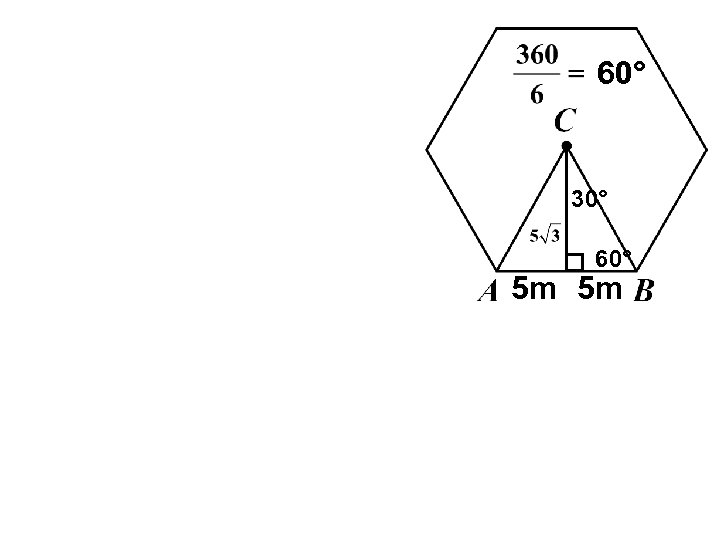60° 30° 60° 5 m 5 m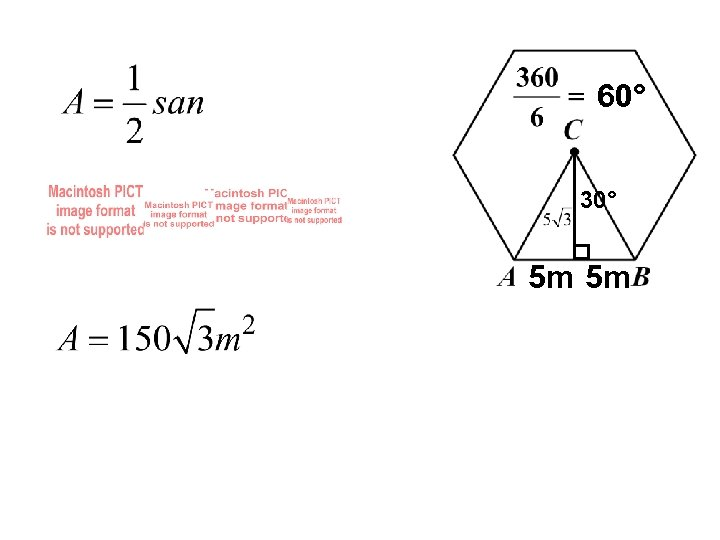60° 30° 5 m 5 m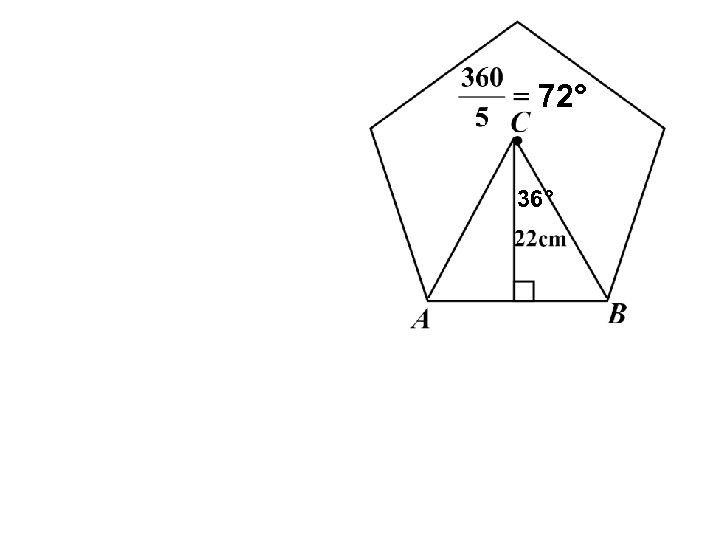72° 36°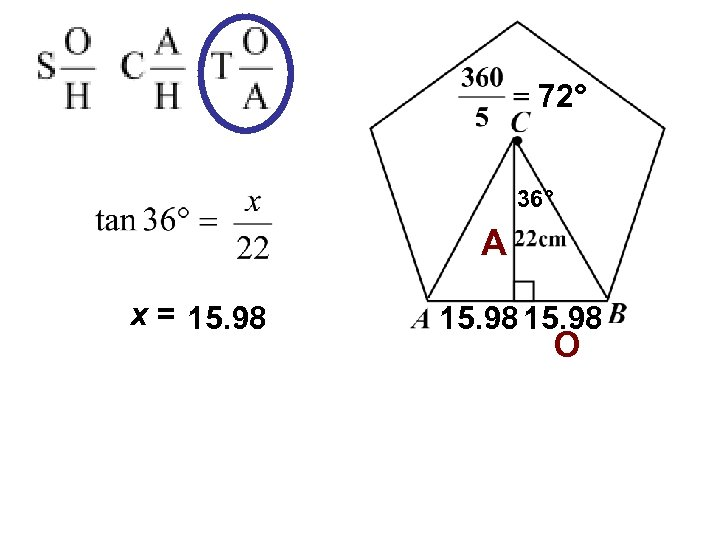72° 36° A x = 15. 98 O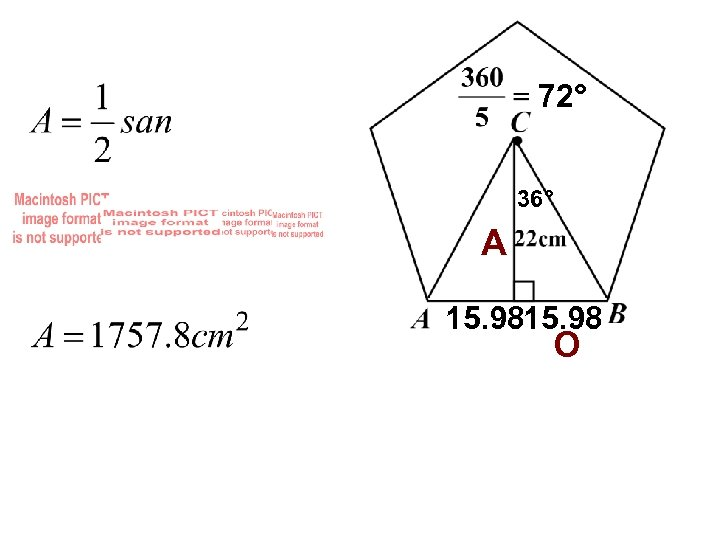72° 36° A 15. 98 O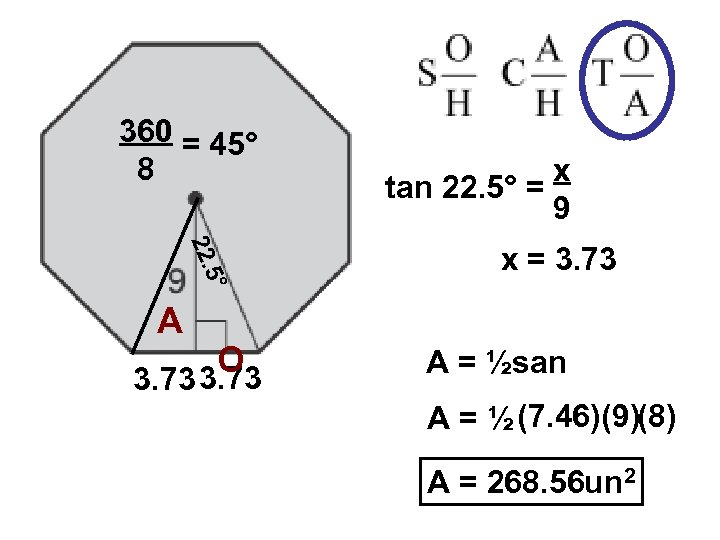360 = 45° 8 x tan 22. 5° = 9 ° 5 22. x = 3. 73 A O 3. 73 A = ½san A = ½ (7. 46)(9)(8) A = 268. 56 un 2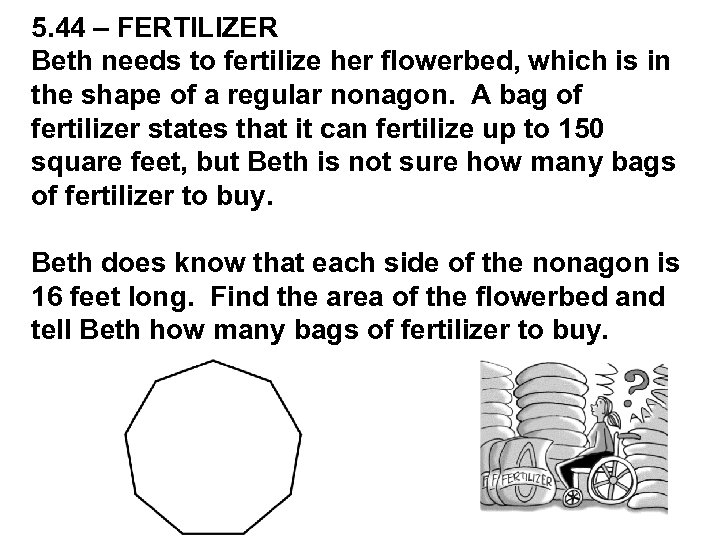5. 44 – FERTILIZER Beth needs to fertilize her flowerbed, which is in the shape of a regular nonagon. A bag of fertilizer states that it can fertilize up to 150 square feet, but Beth is not sure how many bags of fertilizer to buy. Beth does know that each side of the nonagon is 16 feet long. Find the area of the flowerbed and tell Beth how many bags of fertilizer to buy.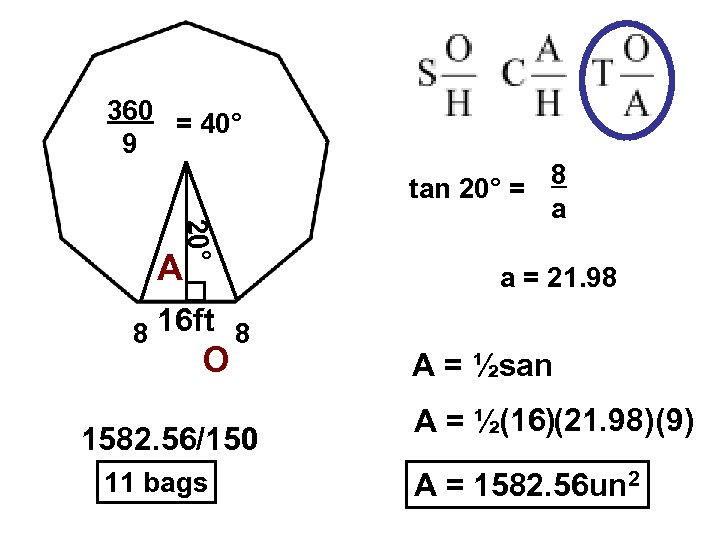360 = 40° 9 tan 20° = 20° A 8 16 ft 8 O 1582. 56/150 11 bags 8 a a = 21. 98 A = ½san A = ½(16)(21. 98)(9) A = 1582. 56 un 2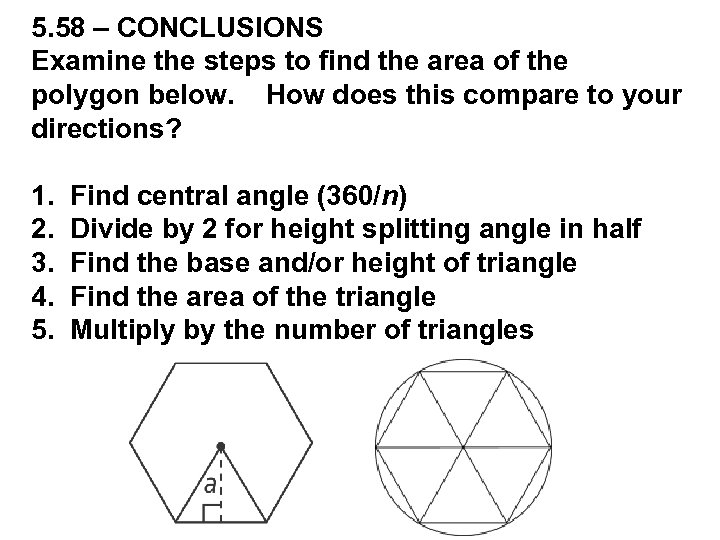5. 58 – CONCLUSIONS Examine the steps to find the area of the polygon below. How does this compare to your directions? 1. Find central angle (360/n) 2. Divide by 2 for height splitting angle in half 3. Find the base and/or height of triangle 4. Find the area of the triangle 5. Multiply by the number of triangles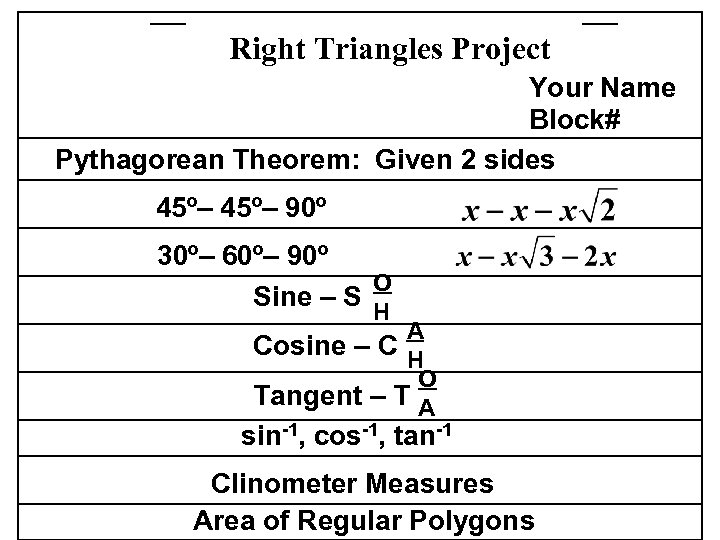Right Triangles Project Your Name Block# Pythagorean Theorem: Given 2 sides 45º– 90º 30º– 60º– 90º O Sine – S H A Cosine – C H O Tangent – T A sin-1, cos-1, tan-1 Clinometer Measures Area of Regular Polygons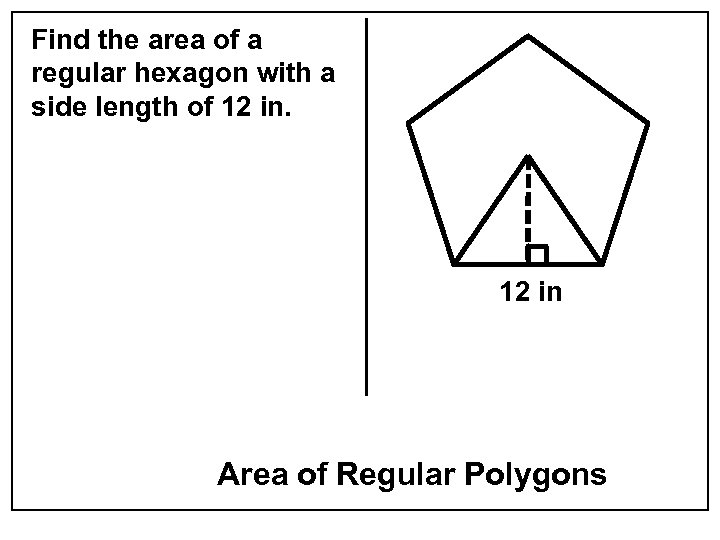Find the area of a regular hexagon with a side length of 12 in Area of Regular Polygons